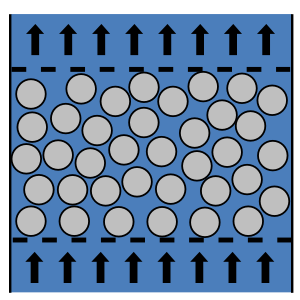# Summary

As fluid flows through a packed bed it experiences a pressure loss due to friction. This article describes the use of the Carman-Kozeny and Ergun equations for the calculation of pressure drop through a randomly packed bed of spheres.

# Definitions

 : Packed bed friction factor : Gravitational constant ( g = 9.81 m/s) : Height of the packed bed (m) : Fluidisation pressure drop (Pa) : Pressure drop through the packed bed (Pa) : Superficial fluid velocity (m/s) : Spherical equivalent particle diameter (m) : Bed voidage : Density of the fluid flowing through the packed bed (kg/m3) : Density of particles in the packed bed (kg/m3) : Viscosity of the fluid flowing through the packed bed (Pa.s)

# Introduction

As a fluid passes through a packed bed it experiences pressure loss due to factors such as friction. The relationships required to predict the pressure drop for a fluid flowing through a packed bed have been known for some time, with Darcy observing in 1896 that the laminar flow of water through a bed of sand was governed by the following relationship:

This relationship was initially analysed in terms of the Hagen-Poiseuille equation for laminar flow through a tube and was later formulated as the Carman-Kozeny equation for pressure drop for laminar flow through a packed bed in 1937. The following sections present the Carman-Kozeny equation and subsequently Ergun’s general equation for the pressure drop through a randomly packed bed of spheres.# Laminar flow through a packed bed

The pressure drop for laminar fluid flow through a randomly packed bed of monosized spheres with diameter may be calculated using the Carman-Kozeny equation as follows:

# Turbulent Flow through a packed bed

The pressure drop for turbulent flow through a packed bed may be calculated from the turbulent component of the Ergun equation (discussed in section 5) as presented below:

# General Equation for pressure drop through a packed bed

Ergun (1952), using a extensive set of experimental data covering a wide range of particle size and shapes, presented a general equation to calculate the pressure drop across a packed bed for all flow conditions (laminar to turbulent). This equation is commonly referred to as the Ergun equation for flow through a randomly packed bed of spheres and takes the following form:

The Ergun equation combines both the laminar and turbulent components of the pressure loss across a packed bed. In laminar flow conditions the first component of the equation dominates with the Ergun equation essentially reducing to the Carman-Koreny equation presented in Section 3, although with a slight variation in the constants used due to variations in the experimental data with which the correlations was developed. In the laminar region the pressure drop through the packed bed is independent of fluid density and has a linear relationship with superficial velocity.

Under turbulent flow conditions the second component of the Ergun equation dominates. Here the pressure drop increases with the square of the superficial velocity and has a linear dependence on the density of the fluid passing through the bed.

## Calculating a Packed Bed Friction Factor

The Ergun equation may also be expressed through the use of a packed bed friction factor in a similar manner to how pressure drop is calculated for fluid flow in a pipe with the Darcy friction factor. The packed bed friction factor may be calculated using the packed bed Reynolds number as follows:

The Ergun equation may then be calculated using the packed bed friction factor as expressed below:

# Packed Bed of Non-Spherical Particles

Although the Ergun equation was constructed for mono-sized spherical particles, pressure drop can still be calculated for randomly packed non-spherical particles using the spherical equivalent particle diameter (the diameter of a sphere having the same surface area to volume ratio as the non-spherical particle). Here the Ergun equation becomes :

Alternatively if the particles in the packed bed are not mono-sized the surface-volume mean diameter , should be used in place of the spherical equivalent particle diameter .

Article Tags

# Subscribe to our mailing list

 Email Name